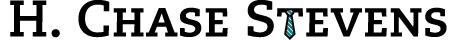# Posts with tag "number theory":

## Fermat's Primality Test

### posted on: Sunday, January 22, 2012 (4:03 pm) by Chase Stevens

Recently, I was reading about some sample interview questions used (at some point) by Google. One such question was "what are the first 10 consecutive digits of e that form a prime number?" Curious, I went to Wikipedia and looked up some primality tests that I could run on 10-digit prime candidates (if you'll forgive the pun) from e. One such test was Fermat's Primality Test, which can be used to probabilistically assess a number's primality (it fails on Carmichael numbers, et alia). I've written up a short python script which runs the test on the numbers 1 to 100 using computed probable primes as possible coprimes and returns all probable primes.

```def test(end):
candidates = range(1,end+1)
primes = list()
for candidate in candidates:
prime = True
for prime in primes:
if candidate % prime:
test_result = ((prime ** (candidate - 1)) % candidate)
print "Candidate: %s, Prime: %s, Result:%s" %(candidate,prime,test_result)
if test_result != 1:
prime = False
break
if prime:
primes.append(candidate)
return primes

test(100)
```

Of course, the astute reader will notice that the above program exhaustively checks each candidate for primality for coprimality with all other probable primes, meaning that even simple trial division would be considerably faster. However, a quick modification to the program that only tested against a fixed number of random probable primes would lead to an increase in efficiency.

Tags: code, math, number theory, probability, programming, python